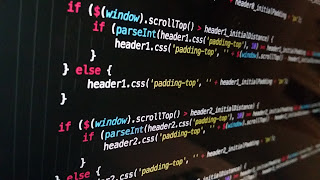# C Programming Print the given no. is odd or even

## C programming print the given no.odd and even

#include<stdio.h>
main()
{
int i;
printf(“Enter a Numbern”);
scanf(“%d”,&i);

###if(i%2==0)

{
printf(“The number %d is Even”,i);

}
else
{
printf(“The number %d is Odd”,i);

}
printf(“n”);

}

## EXPLANATION

Enter a Number
5
The number 5 is Odd

Explanation:
Here we declared an integer ‘i’.

1. Then we took the input from user using scanf statement.
2. As we know modulus operator is used to return the reminder of two values as(10%3=1, 12%2=0).
3. So if you do modulus with 2 then the reminder would be ‘zero’ for Even numbers and ‘1’ for Odd numbers.
4. If we divide ‘i’ with ‘2’ using mod operator then depending upon condition the respective ‘printf’ will be executed.